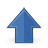# 5. Customizing the Tree genotype

(This part of the documentation presumes you have already studied the basic usage of Tree genotype, described in the Symbolic regression example in Tutorial)

### 1. Types of terminals (in-built)

Terminals in Tree are of type double by default, but may be any of the following types: DOUBLE, INT, BOOL, CHAR, STRING. The type is determined with type name before series of terminals, e.g.:

`<Entry key="terminalset">DOUBLE X Y INT a b c BOOL active</Entry>`

NOTE: currently no type control is implemented in ECF (strongly-typed GP is planned), so the terminals and functions used should be of the same type (or the user-defined type, see example below).

### 2. Defining constants

Terminals of constant values are defined simply by listing them in the "terminalset" parameter. Here the following rule applies: the constant's name is its value.

`<Entry key="terminalset">X Y 0 1 3.141592 BOOL true false</Entry>`

Alternatively, the user can introduce a variable terminal (e.g. with the name "PI"), define its value at the begining (EvaluationOp::initialize) and simply not change it during the evolution.

### 3. Defining Ephemereal random constants (ERCs)

ERCs of in-built types may be defined in the configuration file in the "terminalset" variable, e.g.:

`<Entry key="terminalset">DOUBLE {1.5 3.14159 42} [-3.14 3.14] INT {1 2 3 4 5} BOOL {true false}</Entry>`

Explanation:

• [<lower_bound> <upper_bound>] will work only for DOUBLE typed ERCs and will create a random double (of uniform distribution) in the defined interval

• {<value1> <value2> ...} will create ERCs which can assume only values stated in the set

ERCs of types other than double assume a specific value when inserted in a new tree and that value remains constant during the evolution. Double-typed ERCs can change value if mutated by Gaussian mutation operator ("mut.gauss" in Tree genotype parameters).

To distinguish ERCs from constants in output, any ERC will have a prefix denoting its type, e.g. D_1.234, I_3

TODO: user-type ERCs

### 4. Adding a user defined function primitive

Tree genotype may accept user defined functional primitives (for an example of a functional primitive structure, see example below or existing primitives in 'tree' folder). Adding a functional primitive is performed before State initialization:

```TreeP tree = (TreeP) new Tree;             // create Tree object
PrimitiveP prim = (PrimitiveP) new Prim;   // create primitive object

The primitive is now added to the existing functions, but will be used only if its name is stated in the "primitiveset" variable in the configuration file.

### 5. Adding custom-type terminals and functions

The user may want to perform tree execution with a custom data type; in that case, both function(s) and primitive(s) of that type must be defined. An example:

```// user-defined type example
struct my_type
{
double v;
bool b;
};

// terminal class of type my_type
class MyTerminal : public Primitives::Primitive
{
public:
my_type value_;

MyTerminal()
{
nArguments_ = 0;
}
void execute(void* result, Tree& tree)
{
my_type& res = *(my_type*)result;
res = value_;
}
void setValue(void* value)
{
value_ = *(my_type*)value;
}
~MyTerminal()
{	}
};

// example function with arguments of my_type
class MyFunc : public Primitives::Primitive
{
public:
MyFunc()
{
nArguments_ = 2;
name_ = "func";
}
void execute(void* result, Tree& tree)
{
my_type first, second;
my_type& func = *(my_type*)result;

getNextArgument(&first, tree);
getNextArgument(&second, tree);

// example calculation
func.b = first.b && second.b;
func.v = first.v + second.v;
}
~MyFunc()
{	}
};```

After this, the defined function and terminal may be added to the Tree genotype:

```TreeP tree = (TreeP) new Tree;

PrimitiveP myFunc = (PrimitiveP) new MyFunc;

PrimitiveP myTerm = (PrimitiveP) new MyTerminal;
myTerm->setName("term");
```<Tree>